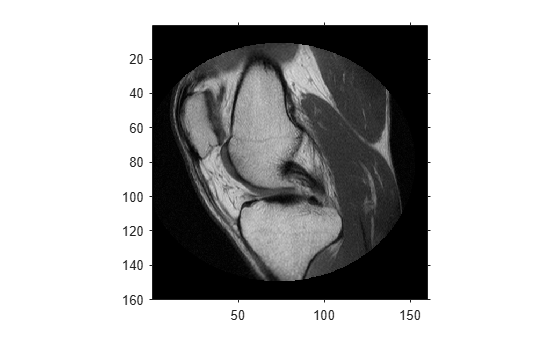# worldToSubscript

Convert world coordinates to row and column subscripts

## Syntax

``[I, J] = worldToSubscript(R,xWorld,yWorld)``
``[I, J, K] = worldToSubscript(R,xWorld,yWorld,zWorld)``

## Description

example

````[I, J] = worldToSubscript(R,xWorld,yWorld)` maps points from the 2-D world system (`xWorld`,`yWorld`) to subscript arrays `I` and `J` based on the relationship defined by 2-D spatial referencing object `R`.If the kth input coordinates (`xWorld`(k),`yWorld`(k)) fall outside the image bounds in the world coordinate system, `worldToSubscript` sets the corresponding subscripts `I`(k) and `J`(k) to `NaN`.```

example

````[I, J, K] = worldToSubscript(R,xWorld,yWorld,zWorld)` maps points from the 3-D world system to subscript arrays `I`, `J`, and `K`, using 3-D spatial referencing object `R`.```

## Examples

collapse all

Read a 2-D grayscale image of a knee into the workspace.

```m = dicominfo('knee1.dcm'); A = dicomread(m);```

Create an `imref2d` object, specifying the size and the resolution of the pixels. The DICOM file contains a metadata field `PixelSpacing` that specifies the image resolution in each dimension in millimeters per pixel.

`RA = imref2d(size(A),m.PixelSpacing(2),m.PixelSpacing(1))`
```RA = imref2d with properties: XWorldLimits: [0.1562 160.1562] YWorldLimits: [0.1562 160.1562] ImageSize: [512 512] PixelExtentInWorldX: 0.3125 PixelExtentInWorldY: 0.3125 ImageExtentInWorldX: 160 ImageExtentInWorldY: 160 XIntrinsicLimits: [0.5000 512.5000] YIntrinsicLimits: [0.5000 512.5000] ```

Display the image, including the spatial referencing object. The axes coordinates reflect the world coordinates. Notice that the coordinate (0,0) is in the upper left corner.

```figure imshow(A,RA,'DisplayRange',[0 512])```Select sample points, and store their world x- and y- coordinates in vectors. For example, the first point has world coordinates (38.44,68.75), the second point is 1 mm to the right of it, and the third point is 7 mm below it. The last point is outside the image boundary.

```xW = [38.44 39.44 38.44 -0.2]; yW = [68.75 68.75 75.75 1];```

Convert the world coordinates to row and column subscripts using `worldToSubscript`.

`[rS, cS] = worldToSubscript(RA,xW,yW)`
```rS = 1×4 220 220 242 NaN ```
```cS = 1×4 123 126 123 NaN ```

The resulting vectors contain the row and column indices that are closest to the point. Note that the indices are discrete, and that points outside the image boundary have `NaN` for both row and column indices.

Also, the order of the input and output coordinates is reversed. The world x-coordinate vector, `xW`, corresponds to the second output vector, `cS`. The world y-coordinate vector, `yW`, corresponds to the first output vector, `rS`.

Read a 3-D volume into the workspace. This image consists of 27 frames of 128-by-128 pixel images.

```load mri; D = squeeze(D); D = ind2gray(D,map);```

Create an `imref3d` spatial referencing object associated with the volume. For illustrative purposes, provide a pixel resolution in each dimension. The resolution is in millimeters per pixel.

`R = imref3d(size(D),2,2,4)`
```R = imref3d with properties: XWorldLimits: [1 257] YWorldLimits: [1 257] ZWorldLimits: [2 110] ImageSize: [128 128 27] PixelExtentInWorldX: 2 PixelExtentInWorldY: 2 PixelExtentInWorldZ: 4 ImageExtentInWorldX: 256 ImageExtentInWorldY: 256 ImageExtentInWorldZ: 108 XIntrinsicLimits: [0.5000 128.5000] YIntrinsicLimits: [0.5000 128.5000] ZIntrinsicLimits: [0.5000 27.5000] ```

Select sample points, and store their world x-, y-, and z-coordinates in vectors. For example, the first point has world coordinates (108,92,52), the second point is 3 mm above it in the +z-direction, and the third point is 5.2 mm to the right of it in the +x-direction. The last point is outside the image boundary.

```xW = [108 108 113.2 2]; yW = [92 92 92 -1]; zW = [52 55 52 0.33];```

Convert the world coordinates to row, column, and plane subscripts using `worldToSubscript`.

`[rS, cS, pS] = worldToSubscript(R,xW,yW,zW)`
```rS = 1×4 46 46 46 NaN ```
```cS = 1×4 54 54 57 NaN ```
```pS = 1×4 13 14 13 NaN ```

The resulting vectors contain the column, row, and plane indices that are closest to the point. Note that the indices are discrete, and that points outside the image boundary have index values of `NaN`.

Also, the order of the input and output coordinates is reversed. The world x-coordinate vector, `xW`, corresponds to the second output vector, `cS`. The world y-coordinate vector, `yW`, corresponds to the first output vector, `rS`.

## Input Arguments

collapse all

Spatial referencing object, specified as an `imref2d` or `imref3d` object.

Coordinates along the x-dimension in the world coordinate system, specified as a numeric scalar or vector.

Data Types: `single` | `double` | `int8` | `int16` | `int32` | `int64` | `uint8` | `uint16` | `uint32` | `uint64`

Coordinates along the y-dimension in the world coordinate system, specified as a numeric scalar or vector. `yWorld` is the same length as `xWorld`.

Data Types: `single` | `double` | `int8` | `int16` | `int32` | `int64` | `uint8` | `uint16` | `uint32` | `uint64`

Coordinates along the z-dimension in the world coordinate system, specified as a numeric scalar or vector. `zWorld` is the same length as `xWorld`.

Data Types: `single` | `double` | `int8` | `int16` | `int32` | `int64` | `uint8` | `uint16` | `uint32` | `uint64`

## Output Arguments

collapse all

Row indices, returned as a positive integer scalar or vector. `I` is the same length as `yWorld`. For an m-by-n or m-by-n-by-p image, 1 ≤ `I`m.

Data Types: `double`

Column indices, returned as a positive integer scalar or vector. `J` is the same length as `xWorld`. For an m-by-n or m-by-n-by-p image, 1 ≤ `J`n.

Data Types: `double`

Plane indices, returned as a positive integer scalar or vector. `K` is the same length as `zWorld`. For an m-by-n-by-p image, 1 ≤ `K`p.

Data Types: `double`

## Version History

Introduced in R2013a